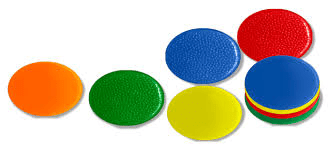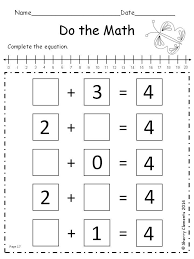# ADDITION OF WHOLE NUMBERS WITH SUM LESS THAN 5

##### Hello children, welcome to class

Add: means to combine two or more numbers to make a larger number. Addition is finding the total, or sum of two or more numbers.

Sum is the result or answer obtained when two or more numbers are added.

Counters are small items we use to help us count and work with numbers.

In adding two numbers together we need counters, examples of counters are matchsticks, beads or buttons.COUNTERS

So when adding we count out the two numbers given, combine them and count all together. Example

2      +     1     =     3

ACTIVITY

1. 2 + 0 =
2. 1 + 3 =
3. 2 + 2 =
4. 0 + 4 =
5. 1 + 2 =

ACTIVITY 2

Count the objects and write down the number you counted.ACTIVITY 3Your Opinion Matters! Quickly tell us how to improve your Learning Experience HERE